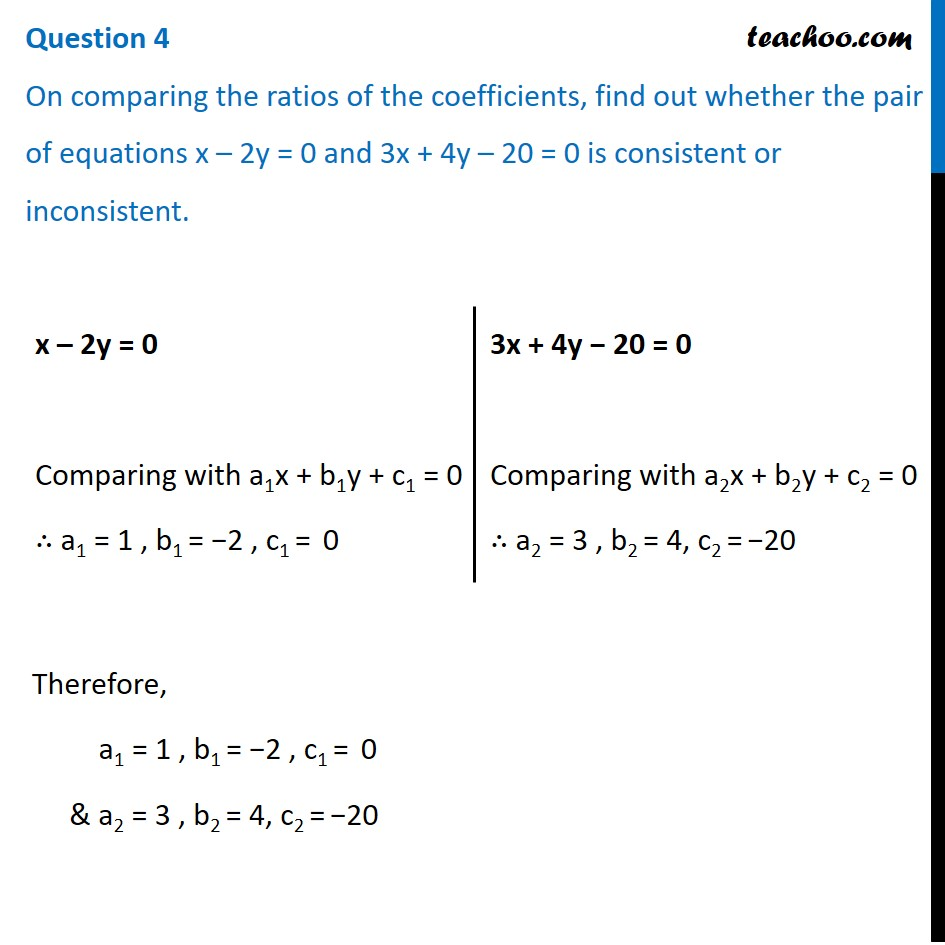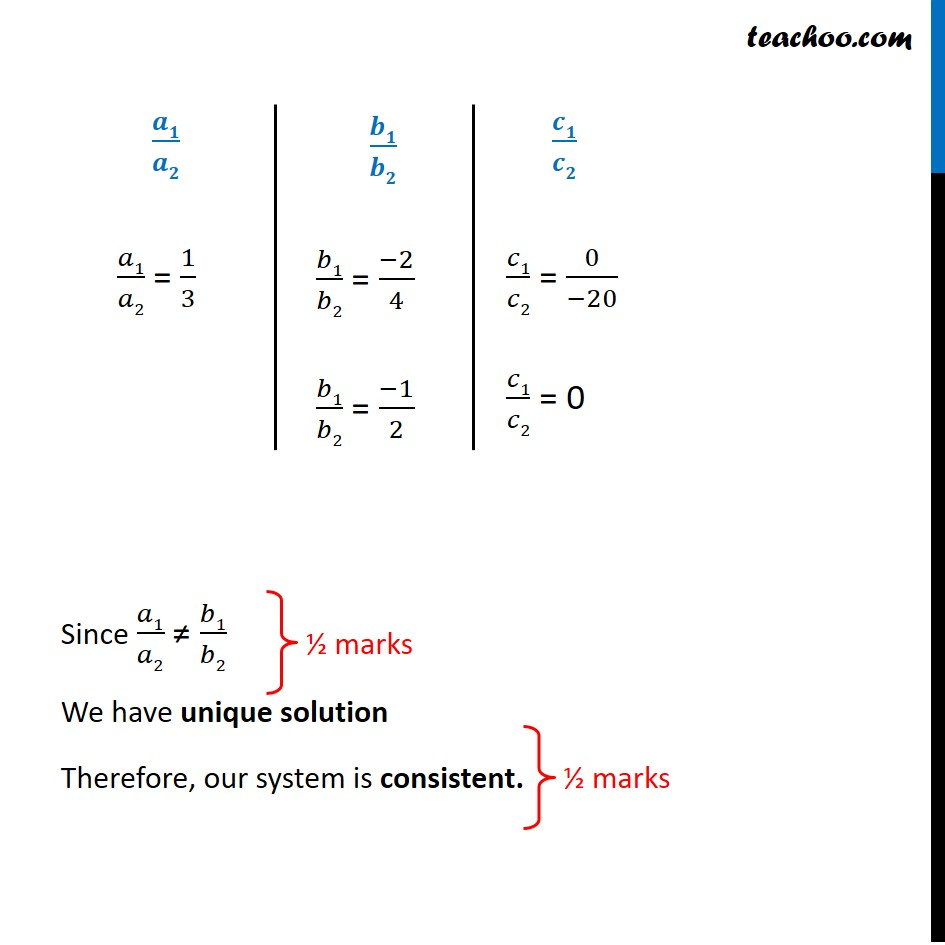CBSE Class 10 Sample Paper for 2021 Boards - Maths Basic

Class 10
Solutions of Sample Papers for Class 10 Boards

## On comparing the ratios of the coefficients, find out whether the pair of equations x – 2y = 0 and 3x + 4y – 20 = 0 is consistent or inconsistent.### Transcript

Question 4 On comparing the ratios of the coefficients, find out whether the pair of equations x – 2y = 0 and 3x + 4y – 20 = 0 is consistent or inconsistent. x – 2y = 0 Comparing with a1x + b1y + c1 = 0 ∴ a1 = 1 , b1 = −2 , c1 = 0 3x + 4y − 20 = 0 Comparing with a2x + b2y + c2 = 0 ∴ a2 = 3 , b2 = 4, c2 = −20 Therefore, a1 = 1 , b1 = −2 , c1 = 0 & a2 = 3 , b2 = 4, c2 = −20 Since 𝑎1/𝑎2 ≠ 𝑏1/𝑏2 We have unique solution Therefore, our system is consistent. 𝑎1/𝑎2 = 1/3 𝑏1/𝑏2 = (−2)/4 𝑏1/𝑏2 = (−1)/2 𝑐1/𝑐2 = 0/(−20) 𝑐1/𝑐2 = 0